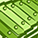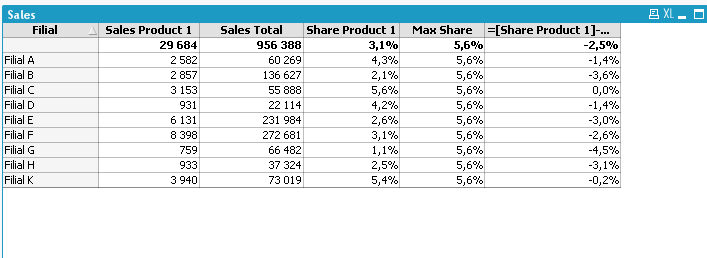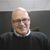Announcements
MAINTENANCE ALERT: Dec. 12th starting 9 AM CET. Community will be read-only. GET DETAILS
cancel
Showing results for
Did you mean:Contributor III

## Maximum value of strings

Hello.

In the column "Share Product 1", I calculate the share of sales of product 1 from sales total for each filial:

Sum ({<Product = {'Product 1'}>}Amount)/Sum (Amount)I need to calculate the maximum share of product 1 among all the filials and the deviation from this share.

1 Solution

Accepted SolutionsChampion

Hi Evgen,

Max( Total aggr(Sum ({<Product = {'Product 1'}>}Amount)/Sum (Amount), Filial))

3 RepliesChampion

Hi Evgen,

Max( Total aggr(Sum ({<Product = {'Product 1'}>}Amount)/Sum (Amount), Filial))Partner - Master

For max share

=max(TOTAL aggr(Sum ({<Product = {'Product 1'}>} Amount)/Sum (Amount), Filial))

Difference

=[Share Product 1]-[Max Share]Partner - Champion III

More for the sake of demonstrating Qlik's flexibility in how to calculate various things I present these two alternative expressions that don't use Aggr() to calculate Max Share:

Sum (TOTAL {<Product={'Product 1'},Filial={"=Rank(Sum({<Product={'Product 1'}>} Amount)/Sum(Amount))=1"}>} Amount) / Sum(TOTAL {<Filial={"=Rank(Sum({<Product={'Product 1'}>} Amount)/Sum(Amount))=1"}>} Amount)

or

RangeMax(Top( [Share Product 1],1,NoOfRows()))

Will both calculate the same as:

Max(TOTAL Aggr(Sum ({<Product = {'Product 1'}>} Amount)/Sum (Amount), Filial))Community Browser